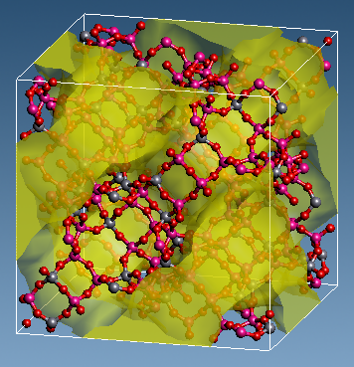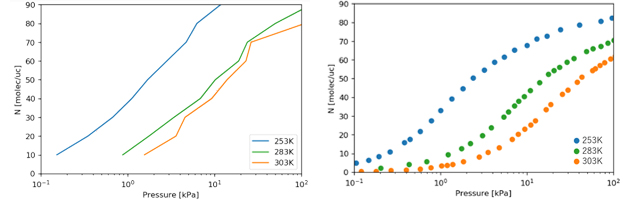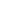Full Atomistic MD
Small molecule penetration / diffusion / adsorption
Materials Science

## Purpose and method

Porous materials such as zeolites and metal-organic-frames (MOFs) are attracting attention for their catalytic functions and gas adsorption properties. In recent years, the reduction of greenhouse gas emissions is necessary, and they are expected to be used for capturing CO2 from the gas mixture.

The adsorption isotherm of gas molecules is a relationship between equilibrium adsorption capacities $$N$$ and gas pressure $$P$$, and is related to the free energy of the gas molecule in the porous materials.
Since the solubility module in J-OCTA can calculate the excess free energy, the pressure $$P$$ of a gas state molecule in equilibrium with $$N$$ molecules adsorbed in zeolite can be calculated using the following equation;

$$\mu$$ex,zeo $$(N)$$ + $$kT$$ ln$$($$$$\rho$$zeo $$(N)$$$$)$$ = $$\mu$$ex,gas + $$\mu_0$$ + $$kT$$ ln $$(P/P_0)$$

$$\mu$$ex: The excess free energy

$$\rho$$zeo: The density of molecules in the zeolite

$$k$$: Boltzmann constant

$$\mu_0$$: The free energy of gas molecules at reference pressure

$$P_0$$: Reference pressure

Zeolite can have a structure in which Si atoms are replaced by Al, and various cations are included to satisfy charge neutrality. Here, we investigated adsorption isotherms for a NaY zeolite (Si/Al ~2.5), which is Na ion containing FAU type zeolite. The force field parameters used in the calculations were obtained from a published study*.Figure 1. Calculation model (Structure of zeolite (NaY) and diffusion region of CO2)

## Simulation result

The adsorption isotherms of CO2 molecules for the NaY zeolite were calculated.
The NaY zeolite model used in the calculations has 54 Al sites, including 54 Na ions. (Figure 1) CO2 molecules move in the region colored yellow in the figure.

Figure 2 shows the calculation results of the adsorption isotherms. The amount of adsorption increased with increasing gas pressure.
We also examined the behavior of the gas at different temperatures, and confirmed that the amount adsorbed tends to decrease with increasing temperature. The absolute value of the adsorption capacity deviated from the experimental results*, but this may be due to that the free energy calculation method is different from that used in the past study.Figure 2. The adsorption isotherms;
(Left) Calculation results (Right) Experimental results*

• Reference
• A. García-Sánchez et al., “Transferable Force Field for Carbon Dioxide Adsorption in Zeolites,” J. Phys. Chem. C, vol. 113, no. 20, pp. 8814–8820, May 2009.This website uses cookies to improve functionality and performance. If you continue browsing the site, you are giving implied consent to the use of cookies on this website. If you want to know more or refuse consent, read our Cookie Policy.

Accept